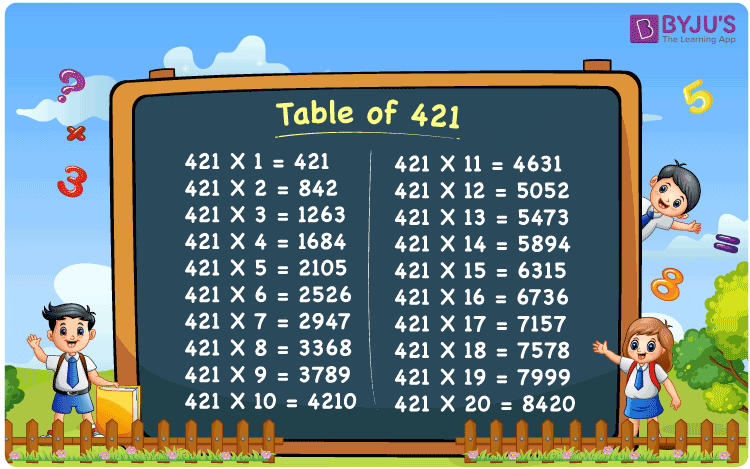Checkout JEE MAINS 2022 Question Paper Analysis : Checkout JEE MAINS 2022 Question Paper Analysis :

# Table of 421

The Table of 421 is the multiplication table which includes values obtained by repeated addition of number 421. For example, to obtain the value of 421 times 5, add 421 for 5 times i.e. 421 + 421 + 421 + 421 + 421 = 2105. The result of 421 times 5 is 2105. Similarly, we can design the table of 421 up to ‘n’ number of times. Learning the multiplication tables helps students to enhance their skills, which are vital from an exam perspective. Students can access the PDF of 421 times table from the link given here.## What is the 421 Times Table?

Multiplication tables are very important in numerical simplifications. Therefore, it is essential to learn the tables for the fast calculation of problems. The below table represents the table of 421 in multiplication and addition form up to 10 times.

 421×1 = 421 421 421×2 = 842 421 + 421 = 842 421×3 = 1263 421 + 421 + 421 = 1263 421×4 = 1684 421 + 421 + 421 + 421 = 1684 421×5 = 2105 421 + 421 + 421 + 421 + 421 = 2105 421×6 = 2526 421 + 421 + 421 + 421 + 421 + 421 = 2526 421×7 = 2947 421 + 421 + 421 + 421 + 421 + 421 + 421 = 2947 421×8 = 3368 421 + 421 + 421 + 421 + 421 + 421 + 421 + 421 = 3368 421×9 = 3789 421 + 421 + 421 + 421 + 421 + 421 + 421 + 421 + 421 = 3789 421×10 = 4210 421 + 421 + 421 + 421 + 421 + 421 + 421 + 421 + 421 + 421 = 4210

## Multiplication Table of 421

Multiplication tables help students to speed up their mental calculations. For this reason, it is essential to learn the tables for fast calculation of problems. Check the multiples of 421 for 20 times from the below chart.

 421 × 1 = 421 421 × 2 = 842 421 × 3 = 1263 421 × 4 = 1684 421 × 5 = 2105 421 × 6 = 2526 421 × 7 = 2947 421 × 8 = 3368 421 × 9 = 3789 421 × 10 = 4210 421 × 11 = 4631 421 × 12 = 5052 421 × 13 = 5473 421 × 14 = 5894 421 × 15 = 6315 421 × 16 = 6736 421 × 17 = 7157 421 × 18 = 7578 421 × 19 = 7999 421 × 20 = 8420

## Solved Example on the Table of 421

Example 1:

Calculate 421 times 16 minus 500.

Solution:

421 times 16 minus 500

= 421 x 16 – 500

= 6736 – 500

= 6236

## Frequently Asked Questions on Table of 421

### What is 17 times of 421?

17 times of 421 is equal to 7157.
421 x 17 = 7157.

### Using the table of 421, find the value of 421 times 15 plus 90 minus 40.

421 times 15 plus 90 minus 40
= 421 x 15 + 90 – 40
= 6315 + 90 – 40
= 6365

### Is 421 a prime number?

Yes, 421 is a prime number.